Courses

# Transformers Class 12 Notes | EduRev

## Class 12: Transformers Class 12 Notes | EduRev

The document Transformers Class 12 Notes | EduRev is a part of the Class 12 Course Physics Class 12.
All you need of Class 12 at this link: Class 12

11. Transformer :

A transformer is an electrical device for converting an alternating current at low voltage into that at high voltage or vice versa. If it increase the input voltage, it is called step up transformer and if it decreases the input voltage, it is called step down transformer.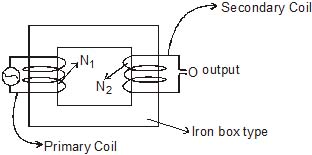Principle : It works on the principle of mutual induction, i.e., when a changing current is passed through one of the two inductively coupled coils, an induced emf is setup in the other coil.

Construction : A transformer essentially consists of two coils of insulated copper wire having different number of turns and wound on the same soft iron core. The coil to which electric energy is supplied is called the primary and the coil from which energy is drawn or output is obtained is called the secondary.

To prevent energy losses due to eddy currents, a laminated sheet is used. Because of high permeability of soft iron, the entire magnetic flux due to the current in the primary coil practically remains in the iron core and hence passes fully through the secondary.

Two types of arrangements are generally used for winding of primary and secondary coils in a transformer.

1. Core type : In this type, the primary and secondary coils are wound on separate limbs of the core.

2. Shell type : In this type the primary and secondary coils are wound one over another on the same limb of the iron core.

Theory : Consider the situation when no load is connected to the secondary, i.e., its terminals are open. Let N1 and N2 be the number of terminal in the primary and secondary respectively. Then Induced emf in the primary coil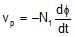Induced emf in the secondary coil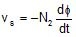where φ is the magnetic flux linked with each turn of the primary or secondary at any instant. Thus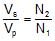The ratio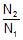of the number of turns in the secondary to that in the primary called the turns ratio of the transformer. It is also called transformation ratio

for step up transformer : N2 > N1

for step down transformer : N1 > N2

Currents in primary and secondary : Assuming the transformer to be ideal one so that there are no energy loss, then

Input power = output power

or Vp Ip = Vs Is

where Ip and Iare the currents in the primary and secondary respectively.

Hence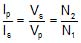Efficiency : The efficiency of a transformer is defined as

η =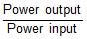× 100%

The efficiency of real transformer is fairly high (90 - 98%) though not 100%

Extra Portion For IIT-Mains

Explain with the help of a labelled diagram, the principle and working of an a.c. generator ? Write the expression for the emf generated in the coil in terms of speed of rotation. Can the current produced by an a.c. generator be measured with a moving coil galvanometer.

Sol. AC generator : A dynamo or generator is a device which converts mechanical energy into electrical energy. It is based on the principle of electromagnetic induction.

Construction : It consists of the four main parts :

(i) Field Magnet : It produces the magnetic field. In the case of a low power dynamo, the magnetic field is generated by a permanent magnet, while in the case of large power dynamo, the magnetic field is produced by an electromagnet.

(ii) Armature : It consists of a large number of turns of insulated wire in the soft iron drum or ring. It can revolve rebound an axle between the two poles of the field magnet. The drum or ring serves the two purposes :

(i) It serves as a support to coils and

(ii) It increases the magnetic field due to air core being replaced by an iron core :

(iii) Slip Rings : The slip rings R1 and R2 are the two metal rings to which the ends of armature coil are connected. These rings are fixed to the shaft which rotates the armature coil so that the rings also rotate along with the armature.

(iv) Brushes : These are two flexible metal plates or carbon rods (B1 and B2) which are are fixed and constantly touch the revolving rings. The output current in external load RL is taken through these brushes.

Working : When the armature coil is rotated in the strong magnetic field, the magnetic flux linked with the coil changes and the current is induced in the coil, its direction being given by Fleming's right hand rule. Considering the armature to be in vertical position and as it rotates

in anticlockwise direction, the wire ab moves upward and cd downward, so that the direction of induced current is shown in fig. In the external circuit, the current flows along B1 BL B2. The direction of current remains unchanged during the first half turn of armature. During the second half revolution, the wire ab moves downward and cd upward, so the direction of current is reversed and in external circuit if flows along B2 RL B1. Thus the direction of induced emf and current changes in the external circuit after each half revolution.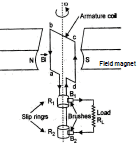If N is the number of turns in coil, f the frequency of rotation. A area of coil and B the magnetic induction, then induced emf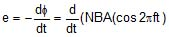Obviously, the emf produced is alternating and hence the current is also alternating

Current produced by an ac generator cannot be measured by moving coil ammeter, because the average value of ac over fully cycle is zero.

The document Transformers Class 12 Notes | EduRev is a part of the Class 12 Course Physics Class 12.
All you need of Class 12 at this link: Class 12Use Code STAYHOME200 and get INR 200 additional OFF Use Coupon Code
All Tests, Videos & Notes of Class 12: Class 12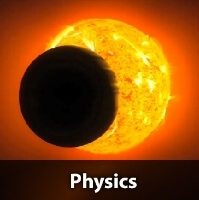## Physics Class 12

132 videos|326 docs|167 tests

Track your progress, build streaks, highlight & save important lessons and more!

,

,

,

,

,

,

,

,

,

,

,

,

,

,

,

,

,

,

,

,

,

;